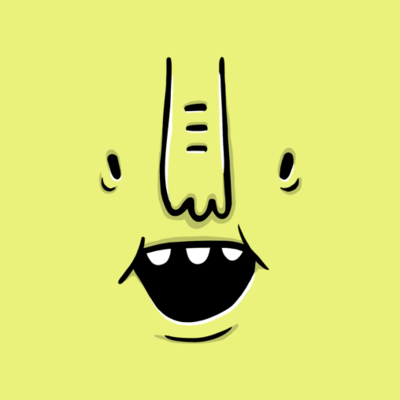## University# Introduction to Set Theory## 13 questions

See Preview
• Multiple Choice
30 seconds
1 pt

What is a set?

A method of solving problems

A collection of objects

A 2-D shape

{7}

• Multiple Choice
30 seconds
1 pt

$\left\{\frac{a}{b}\left|a,b\in Z;b\ne0\right|\right\}\$  defines what basic set?

Reals

Naturals

Rationals

Integers

• Multiple Choice
30 seconds
1 pt

What is the following symbol's name and meaning? $\cup$

Union- Combines two sets or intervals

Union- Gives the overlap of two sets or intervals

Intersection- Combines 2 sets or intervals

Intersection- Gives the overlap of two sets or intervals